Next: Two-State System Up: Time-Independent Perturbation Theory Previous: Introduction

# Improved Notation

Before commencing our investigation, it is helpful to introduce some improved notation. Let the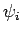be a complete set of eigenstates of the Hamiltonian,, corresponding to the eigenvalues: i.e.,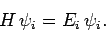(855)

Now, we expect theto be orthonormal (see Sect. 4.9). In one dimension, this implies that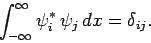(856)

In three dimensions (see Cha. 7), the above expression generalizes to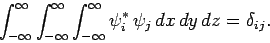(857)

Finally, if theare spinors (see Cha. 10) then we have(858)

The generalization to the case where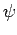is a product of a regular wavefunction and a spinor is fairly obvious. We can represent all of the above possibilities by writing(859)

Here, the term in angle brackets represents the integrals in Eqs. (856) and (857) in one- and three-dimensional regular space, respectively, and the spinor product (858) in spin-space. The advantage of our new notation is its great generality: i.e., it can deal with one-dimensional wavefunctions, three-dimensional wavefunctions, spinors, etc.

Expanding a general wavefunction,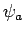, in terms of the energy eigenstates,, we obtain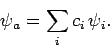(860)

In one dimension, the expansion coefficients take the form (see Sect. 4.9)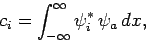(861)

whereas in three dimensions we get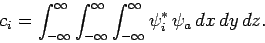(862)

Finally, ifis a spinor then we have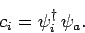(863)

We can represent all of the above possibilities by writing(864)

The expansion (860) thus becomes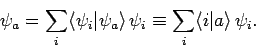(865)

Incidentally, it follows that(866)

Finally, ifis a general operator, and the wavefunctionis expanded in the manner shown in Eq. (860), then the expectation value ofis written (see Sect. 4.9)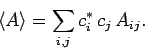(867)

Here, the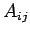are unsurprisingly known as the matrix elements of. In one dimension, the matrix elements take the form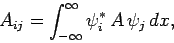(868)

whereas in three dimensions we get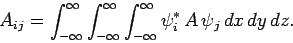(869)

Finally, ifis a spinor then we have(870)

We can represent all of the above possibilities by writing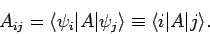(871)

The expansion (867) thus becomes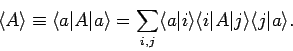(872)

Incidentally, it follows that [see Eq. (194)]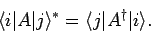(873)

Finally, it is clear from Eq. (872) that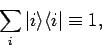(874)

where theare a complete set of eigenstates, and 1 is the identity operator.Next: Two-State System Up: Time-Independent Perturbation Theory Previous: Introduction
Richard Fitzpatrick 2010-07-20